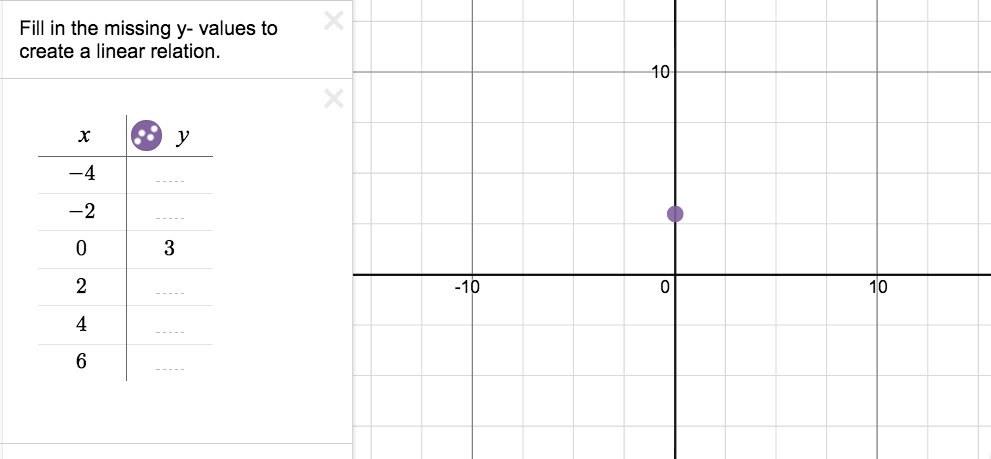Home > High School: Functions > Line Builders

# Line Builders

Directions: Complete the table & graph belowor here on Desmos to create a linear relation. Find the equation of the linear relation.

Fill in the table again and again to create as many different linear relations as you can.
What do the graphs have in common? What do the equations have in common?

### Hint

You get to create any pattern you like….put one point in. What pattern have you started between the points?

### Answer

possible answers (infinite):
y = 3x + 3
y = 1/2x + 3
y = 2x + 3
y = -2x + 3

The graphs all have the same y-intercept, and therefore have the same constant.

Source: Jon Orr

## Creating Sequences

Directions: Using the digits 0-9, at most one time each, complete the first three terms …